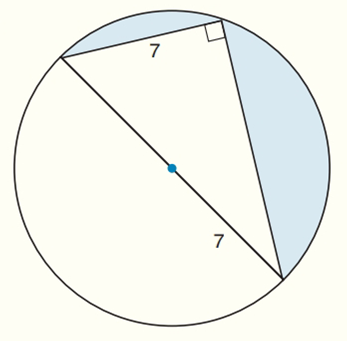Chapter 8.CR, Problem 28CR### Elementary Geometry for College St...

6th Edition
Daniel C. Alexander + 1 other
ISBN: 9781285195698

#### Solutions

Chapter
Section### Elementary Geometry for College St...

6th Edition
Daniel C. Alexander + 1 other
ISBN: 9781285195698
Textbook Problem
1 views

# Find the exact areas of the shaded regions in Exercises 27 to 31.To determine

To find:

The exact areas of the shaded regions.

Explanation

Calculation:

Sketch the following diagram:

The area of right angle triangle A=12bh

To find height:

The given triangle is 30-60-90-degree right triangle.

Long leg of the triangle = short leg ×3

To find short leg (a) of the triangle:

2a=142a2=142a=7

Long leg of the triangle (a3) = 73

Height (h) = 73 and base (b) = 7

To find the area of semi-circle:

Radius (r) = OC = 7

### Still sussing out bartleby?

Check out a sample textbook solution.

See a sample solution

#### The Solution to Your Study Problems

Bartleby provides explanations to thousands of textbook problems written by our experts, many with advanced degrees!

Get Started

#### Evaluate the expression sin Exercises 116. (32)3

Finite Mathematics and Applied Calculus (MindTap Course List)

#### Use an integral to estimate the sum i=110000i.

Single Variable Calculus: Early Transcendentals, Volume I

#### For , f′(x) =

Study Guide for Stewart's Single Variable Calculus: Early Transcendentals, 8th

#### Find for defined implicity by .

Study Guide for Stewart's Multivariable Calculus, 8th